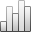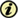Acta Stereologica

0351-580XMore Statisticssince 05 December 2013 :
View(s): 165 (1 ULiège)
Yang Zhengwei, Liu Guoquan & Nigel G. Wreford

### A new distance method for the quantitative analysis of point patterns in space

Article#### Abstract

A simple way to analyze the spatial distribution of a given point set in space is to first measure the nearest neighbor distance (the distance from a random point sampled to its nearest neighbor point). Then a completely random point pattern with the same point density is generated by computer simulation (a Poisson point process) and the nearest neighbor distances measured in the same way. By comparing the sizes and distributions of the distances, the pattern [clustered, random, or systematic (regular)] of the given point set is determined. It is demonstrated in this paper that the complex and time-consuming procedure, computer simulation of a random point set, can be replaced by a simpler measurement of another nearest distance - the nearest star distance. This is the distance from a random test point "thrown" into the space to its nearest neighbor point from the given point set. A mean nearest neighbor distance which is smaller than, the same as or bigger than the mean nearest star distance, in combination with their distribution, will suggest a clustered, random or systematic point pattern, respectively.

Keywords : point pattern, spatial distribution, stereology

Yang Zhengwei, Liu Guoquan & Nigel G. Wreford, «A new distance method for the quantitative analysis of point patterns in space», Acta Stereologica [En ligne], Volume 17 (1998), Number 2 - Sep. 1998, 265-272 URL : https://popups.ulg.ac.be/0351-580x/index.php?id=2641.